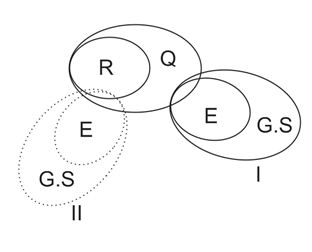# SSC CGL EXAMS 2019 | Reasoning Ability Practice Questions (Day-24)

Dear Aspirants, Here we have given the Important SSC Exam 2019 Practice Test Papers. Candidates those who are preparing for SSC 2019 can practice these questions to get more confidence to Crack SSC 2019 Examination.

[WpProQuiz 5078]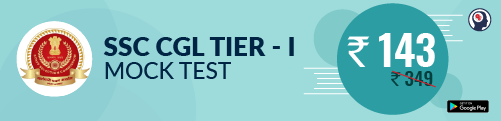1) In a certain code language “my aim is” written as “#@” and “your aim was” written as ”\$©”. “His Aim was” written as “\$¥”. In the same code language, “Her aim was” written as.

a) * \$

b) **

c) \$

d) None of these

2) Find the related letter/ word/ number from the given alternatives:

Psychiatrist: Human Psychology : : Botany : ?

a) Xylem

b) Phloem

c) Xylophone

d) Both (a) & (b)

3) If * denotes, # denotes +, \$ denotes  and Ó denotes —, then 20 * 10 # 30 Ó 40 \$ 8?

a) – 297

b) +297

c) 288

d) 348

4) In a certain code, “STUDY” is written as ‘TVXHD’ on the same logic ‘PLANET’ is written as–

a) REOJZG

b) ROREZJ

c) Cannot be determine

d) ROERJZ

5) Find the odd number letters/ number pair from the given alternatives –

a) 62

b) 42

c) 12

d) 82

7) If ‘PLUTO’ is written as ‘004’, what will be ‘VARUN’–?

a) 4201

b) 1024

c) 5422

d) 2254

8) Select the missing number from the given expenses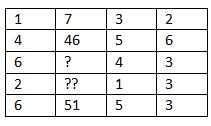a) 48, 7

b) 7, 48

c) 13, 56

d) 15, 24

7) If ‘PLUTO’ is written as ‘004’, what will be ‘VARUN’–?

a) 4201

b) 1024

c) 5422

d) 2254

8) Select the missing number from the given expenses

a) 48, 7

b) 7, 48

c) 13, 56

d) 15, 24

9) Arrange the given words are ascending order as they are in English dictionary.

Pick

Piety

Pesto

Pin

Pirated

Prated

a) C, A, B, E, F, D

b) D, C, B, E, F, A

c) C, A, B, F, E, D

d) C, A, B, D, E, F

10)  In the following question below are given some statements followed by some conclusions. Taking the given statements to be true even if they seem to be at variance from commonly known facts, read all the conclusions and then decide which of the given conclusion logically follows the given statements

Statements:

I. All Reasoning is quant

II. Some Quant is English

III. All English is G.S

Conclusion:

I. Some Reasoning is English

II. Some quant is G.S

III. Some G.S is Quant

a) Only I & II true

b) Only II & III true

c) All are true

d) None of these

My Aim is = ∆#@

His aim was = ∆\$¥

Aim = ∆         Is = @        His = ¥

Her aim was = *\$

Human psychology is related to a psychiatrist and Botany is subject where we study about xylem & phloem.

20 ÷ 10 + 30 – 40 × 8

= 2 + 30 – 320

= 23 – 320 = – 297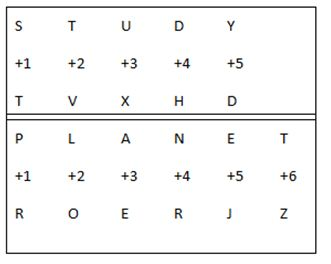82 is odd because sum of digit of 82 is = 10 which is double digits number where 62, 42 and 12 having single digit of sum odd digit as 8, 6 and 3 respectively.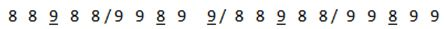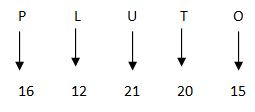Difference of alternate numbers

= (16 + 21 + 15) – (12 + 20)

= 52 – 32

= 202 = 400

= 004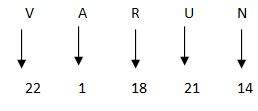Difference of alternate numbers

= (22+18+14) – (1+21)

= (54– 22)

= 322  = 1024

= 4201

12 + 3 2 = 7; 42 + 5  6 = 46:

62 + 4  3 = (48) ; 22 + 1 3 = 7;

62 + 5  3 = 36 + 15 = 51

C. Pesto

A. Pick

B. Piety

D. Pin

E. Pirated

F. Prated